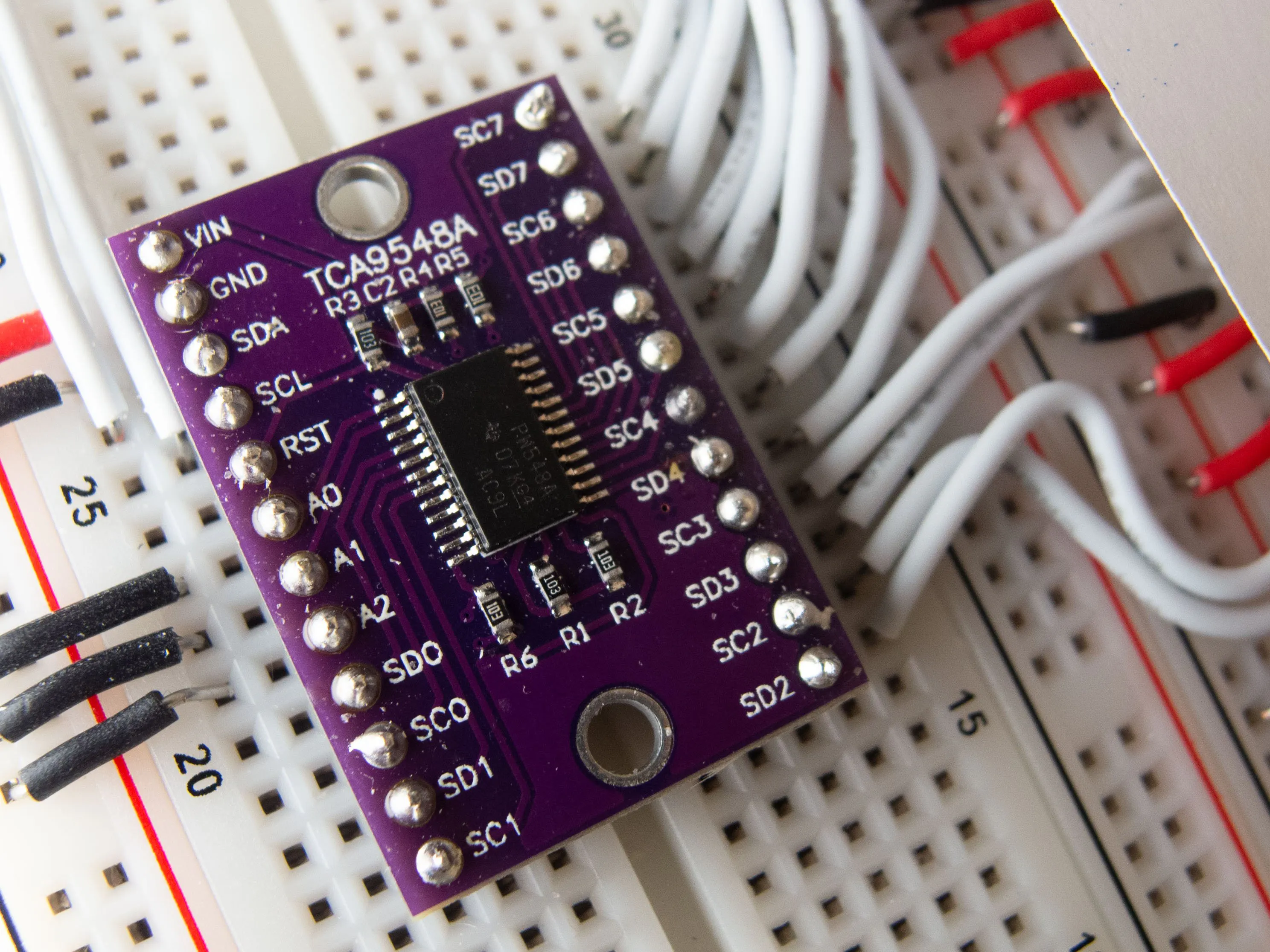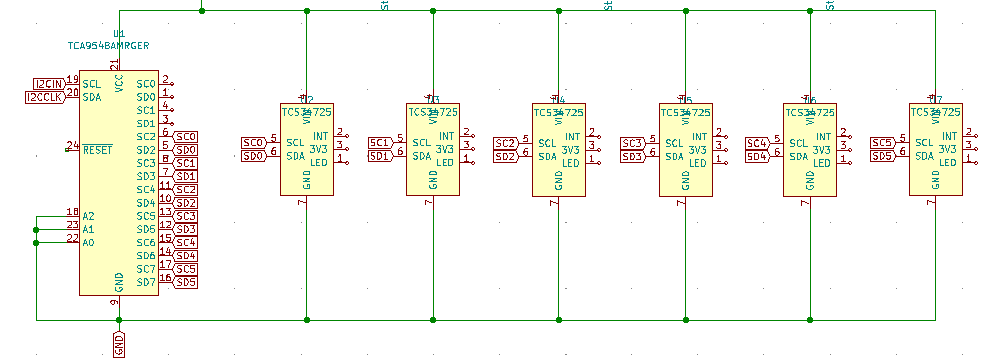# Multiplexing 6 I2C TCS34725 Color Sensors © GPL3+

Have you ever had to use sensors that use the I2C protocol, but realize that they all have the same, non-changeable address?

• 251 views
• 2 respects

## Apps and online services

While working on a project including multiple colors (which I hope to post soon), I realized I needed to use some sort of color sensor/camera. I decided to use 6 TCS34725 modules, which will be able to return the RGB values of certain colors to me. The problem was that these TCS34725 modules use the exact same address: 0x29! This was a problem for me since I needed 6 of these modules, and since the sensors all have the same address, it won't know which ones which! This is when I realized I can use a multiplexer to get these sensor signals into one.

Here, I decided to use an 8-to-1 TCA9548A multiplexer. This has an I2C address of 0x70-0x77, meaning theoretically you can have up to 64 devices on this multiplexer! That's crazy!

For now, I put an RGB LED and a button which will output the RGB values a sensor reads. This is so that when I show a specific sensor a color, it can tell me what the exact color is. For the real project, these RGB value would be used for processing and used for a more complex algorithm. I also used a gamma table to make it closer to how we would see it, and used the clear value to filter out any IR coming through.

It is a little bit inaccurate for now, but it is good enough for my purposes! It most likely has to due with how close I made these sensors in the first place. Hopefully I can show you my new project soon!

## Code

##### tcs34725.inoC/C++
```// Setting up 6 TCS34725 sensors together and reading their output values
// TCA9548A

#include <Wire.h>

#define  inPin  7
#define  rPin   8
#define  gPin   9
#define  bPin   10

byte count = 0;
int currentTime = 0;

char data = {{'r', 'g', 'b'},
{'r', 'g', 'b'},
{'r', 'g', 'b'},
{'r', 'g', 'b'},
{'r', 'g', 'b'},
{'r', 'g', 'b'}};
byte gammatable;

void setup(){
Serial.begin(9600);
Wire.begin();
pinMode(inPin, INPUT_PULLUP);
pinMode(rPin, OUTPUT);
pinMode(gPin, OUTPUT);
pinMode(bPin, OUTPUT);
// gammatable for more color accuracy when outputting on LED
for(int i =0; i < 256; i++){
float x = i;
x /= 255;
x = pow(x, 2.5);
x *= 255;
gammatable[i] = x;
}
initColorSensors();
attachInterrupt(digitalPinToInterrupt(inPin), changeLED, CHANGE);
}
void loop(){
//for(int i = 0; i < sizeof(data); i++){ // get all colors... not necessary right now
//}
for(int i = 0; i < 5; i++){
}

}
void changeLED(){
if(millis() - currentTime > 200){
count++;
currentTime = millis();
}
if(count > 5){
count = 0;
}

}
void initColorSensors(){
for(int i = 0; i < 6; i++){
Serial.println(i);
chooseBus(i);
if (tcs[i].begin()){
Serial.print("Found sensor "); Serial.println(i+1);
} else{
Serial.println("No Sensor Found");
while (true);
}
}
}
chooseBus(sensorNum);
uint16_t r, g, b, c;
tcs[sensorNum].getRawData(&r, &g, &b, &c); // reading the rgb values 16bits at a time from the i2c channel
processColors(r, g, b, c); // processing by dividng by clear value and then multiplying by 256
//data[sensorNum] = r;
//data[sensorNum] = g;
//data[sensorNum] = b;
Serial.print("R: "); Serial.print(r, DEC); Serial.print(" ");
Serial.print("G: "); Serial.print(g, DEC); Serial.print(" ");
Serial.print("B: "); Serial.print(b, DEC); Serial.print(" "); Serial.print(c); Serial.print(" ");
displayLED(r, g, b);
Serial.println(sensorNum);
}
void processColors(uint16_t r, uint16_t g, uint16_t b, uint32_t c){
// getting rid of IR component of light
r /= c;
g /= c;
b /= c;
r *= 256;
g *= 256;
b *= 256;
}

void chooseBus(uint8_t bus){
Wire.beginTransmission(0x70);
Wire.write(1 << (bus+2)); // will be using 2-7 instead of 0-5 because of convience (placed better on the breadboard)
Wire.endTransmission();
}
void displayLED(uint16_t r, uint16_t g, uint16_t b){
if (r > 255 || g > 255 || b > 255){
analogWrite(rPin, 0);
analogWrite(gPin, 0);
analogWrite(bPin, 0);
} else{
analogWrite(rPin, gammatable[(int)r]);
analogWrite(gPin, gammatable[(int)g]);
analogWrite(bPin, gammatable[(int)b]);
}

}
```

## SchematicsMarch 6, 2021

#### Members who respect this project

See similar projects
you might like

#### Connect OLED Screens and I2C Sensors Over 100ft Away!

Project tutorial by Christian

• 4,649 views
• 1 comment
• 9 respects

• 37,037 views
• 41 respects

• 21,858 views
• 39 respects

#### Musical Color

Project tutorial by juraspb

• 11,172 views